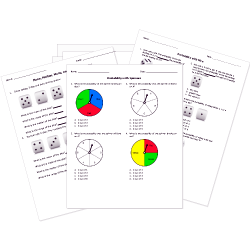Looking for Statistics worksheets?
Check out our pre-made Statistics worksheets!
 Tweet##### Browse Questions

You can create printable tests and worksheets from these Grade 10 Collecting and Interpreting Data questions! Select one or more questions using the checkboxes above each question. Then click the add selected questions to a test button before moving to another page.

Previous Next
Grade 10 Collecting and Interpreting Data CCSS: HSS-CP.A.1
For the sets $A = {green, yellow, blue, red}$ and $B = {blue}$, find $AuuB$.
1. ${blue}$
2. ${green, yellow, red}$
3. ${green, yellow, blue, red}$
4. $emptyset$
Grade 10 Collecting and Interpreting Data CCSS: HSS-CP.A.2
Grade 10 Collecting and Interpreting Data CCSS: HSS-CP.A.1
Find $A nn B nn C$ if $A = {2,4,6,8,10}, \ \ B = {1,2,3,4,5,6}$, and $C = {2,6,10,14}$.
1. $emptyset$
2. ${2,4,6,10}$
3. ${1,2,3,4,5,6,8,10,14}$
4. ${2,6}$
Grade 10 Collecting and Interpreting Data CCSS: HSS-CP.A.1
Given $A = {1,2,3,4,5}, \ \ B = {4,5,6,7,8}, \ \ C = {6,7,8,9,10}$, find $A uu B uu C$.
1. ${1,2,3,4,5,6,7,8,9,10}$
2. $emptyset$
3. ${4,5,6,7,8}$
4. ${1,2,3,9,10}$
Grade 10 Collecting and Interpreting Data CCSS: HSS-CP.A.1
Find $S_1 nn S_2$ if $S_1 = {a,g,j,t,e,w,z}$ and $S_2 = {b,c,g,t,l,r,e,x,h,p}$.
1. ${a,g,i,t,e,w,z,b,c,g,t,l,r,e,x,h,p}$
2. ${a,b,c,e,g,h,i,l,p,r,t,w,x,z}$
3. ${a,b,c,g,h,i,l,p,r,w,x,z}$
4. ${e,g,t}$
Grade 10 Collecting and Interpreting Data CCSS: HSS-CP.A.1
Let $A = {"numbers greater than 5"}$ and $B= {"numbers less than 12"}$. What is $A nn B ?$
1. ${"numbers between 5 and 12"}$
2. ${"all numbers"}$
3. ${"numbers less than 5 and greater than 12"}$
4. ${5,12}$
Grade 10 Collecting and Interpreting Data CCSS: HSS-CP.A.1
Given $A = {22,24,26,28}, \ \ B = {21,23,25,27}$, find $A nn B$.
1. ${21,22,23,24,25,26,27,28}$
2. $emptyset$
3. ${22,23,24,25,26,27}$
4. ${24,25}$
Grade 10 Collecting and Interpreting Data
Grade 10 Collecting and Interpreting Data CCSS: HSS-CP.A.2
Grade 10 Collecting and Interpreting Data CCSS: HSS-IC.A.1
A research group wants to find out the average number of people in America who do not eat meat. They do surveys of 18 people at a vegetarian restaurant and find out that 90.5% of Americans do not eat meat.
1. The study is too small to be accurate.
2. The study was large enough to be very accurate.
3. The study was not accurate because it surveyed customers of a single type of food outlet.
4. Both A and C.
Grade 10 Collecting and Interpreting Data
Previous Next
You need to have at least 5 reputation to vote a question down. Learn How To Earn Badges.